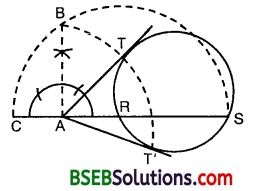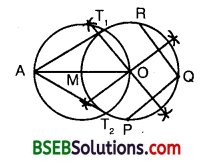# HSSlive: Plus One & Plus Two Notes & Solutions for Kerala State Board

## BSEB Class 10 Maths Chapter 11 Constructions Ex 11.2 Textbook Solutions PDF: Download Bihar Board STD 10th Maths Chapter 11 Constructions Ex 11.2 Book AnswersBSEB Class 10 Maths Chapter 11 Constructions Ex 11.2 Textbook Solutions PDF: Download Bihar Board STD 10th Maths Chapter 11 Constructions Ex 11.2 Book Answers

BSEB Class 10th Maths Chapter 11 Constructions Ex 11.2 Textbooks Solutions and answers for students are now available in pdf format. Bihar Board Class 10th Maths Chapter 11 Constructions Ex 11.2 Book answers and solutions are one of the most important study materials for any student. The Bihar Board Class 10th Maths Chapter 11 Constructions Ex 11.2 books are published by the Bihar Board Publishers. These Bihar Board Class 10th Maths Chapter 11 Constructions Ex 11.2 textbooks are prepared by a group of expert faculty members. Students can download these BSEB STD 10th Maths Chapter 11 Constructions Ex 11.2 book solutions pdf online from this page.

## Bihar Board Class 10th Maths Chapter 11 Constructions Ex 11.2 Books Solutions

 Board BSEB Materials Textbook Solutions/Guide Format DOC/PDF Class 10th Subject Maths Chapter 11 Constructions Ex 11.2 Chapters All Provider Hsslive

## How to download Bihar Board Class 10th Maths Chapter 11 Constructions Ex 11.2 Textbook Solutions Answers PDF Online?

2. Click on the Bihar Board Class 10th Maths Chapter 11 Constructions Ex 11.2 Answers.
3. Look for your Bihar Board STD 10th Maths Chapter 11 Constructions Ex 11.2 Textbooks PDF.
4. Now download or read the Bihar Board Class 10th Maths Chapter 11 Constructions Ex 11.2 Textbook Solutions for PDF Free.

Find below the list of all BSEB Class 10th Maths Chapter 11 Constructions Ex 11.2 Textbook Solutions for PDF’s for you to download and prepare for the upcoming exams:

## BSEB Bihar Board Class 10th Maths Solutions Chapter 11 Constructions Ex 11.2

Question 1.
Draw a circle of radius 6 cm. From a point 10 cm away from its centre, construct the pair of tangents to the circle and measure their lengths.
Solution:
Steps of Construction :
1. Take a point 0 as centre and draw a circle of radius 6 cm.
2. Mark a point P at a distance of 10 cm from the centre O.3. Join OP and bisect it. Let M be its mid-point of OP.
4. With M as centre and MP as radius, draw a circle to intersect the previous circle at Q and R.
5. Join PQ and PR. Then, PQ and PR are the required tangents.
On measurement, we find that PQ = PR = 8 cm.

Justification :
On joining OQ, we find that ∠PQO = 90°, as ∠PQO is the angle in a semi-circle.
∴ PQ ⊥ OQ.
Since OQ is the radius of the given circle, so PQ has to be a tangent to the circle. Similarly, PR is also a tangent to the circle.

Question 2.
Construct a tangent to a circle of radius 4 cm from a point on the concentric circle of radius 6 cm and measure its length. Also, verify the measurement by actual calculation.
Solution:
Steps of Construction :
1. Take a point O and draw two concentric circles of radii 4 cm and 6 cm respectively.
2. Mark a point P on the bigger circle.3. Join OP and bisect it. Let M be its mid-point.
4. With M as centre and MP as radius, draw a circle to intersect the smaller circle at Q and R.
5. Join PQ and PR. Then, PQ and PR are the required tangents.
On measuring, we find that PQ = PR = 4.5 cm (approx.).
Calculation : From A OQP,
OP² = OQ² + PQ²
or 6² = 4² + PQ²
or PQ² = 36 – 16 = 20
So, PQ = 20‾‾‾√cm = 4-47 cm (approx.)
Similarly, PR = 4.47 cm (approx.).

Justification :
On joining OQ, we find that ∠PQO = 90°, as ∠PQO is the angle in a semi-circle.
∴ PQ ⊥ OQ.
Since OQ is the radius of the given circle, so PQ has to be a tangent to the circle. Similarly, PR is also a tangent to the circle.

Question 3.
Draw a circle of radius 3 cm. Take two points P and Q on one of its extended diameter each at a distance of 7 cm from its centre. Draw tangents to the circle from these two points P and Q.
Solution:
Steps of Construction :
1. Take a point O, draw a circle of radius 3 cm with this point as centre.
2. Take two points P and Q on one of its extended diameter such that OP = OQ = 7 cm.
3. Bisect OP and OQ. Let their respective mid-points be M1 and M2.
4. With M1 as centre and M1P as radius, draw a circle to intersect the circle at T1 and T2.5. Join PT1 and PT2. Then, PT1 and PT2 are the required tangents.
Similarly, the tangents QTS and QT4 can be obtained.

Justification :
On joining OT4, we find ∠PT10 = 90°, as an angle in a semi-circle.
∴ PT1 ⊥ OT1
Since OT1 is a radius of the given circle, so PT1 has to be a tangent to the circle.
Similarly, PT2, QT3 and QT4 are also tangents to the circle.

Question 4.
Draw a pair of tangents to a circle of radius 5 cm which are inclined to each other at an angle of 60°.
Solution:
Steps of Construction :
1. With O as centre and radius = 5 cm, draw a circle.
2. Draw any diameter AOC.3. Draw a radius OL such that ∠COL = 60° (i.e., the given angle).
4. At L, draw LM ⊥ OL.
5. At A, draw AN ⊥ OA.
6. These two perpendiculars intersect each other at P. Then, PA and PL are the required tangents.

Justification :
Since OA is the radius, so PA has to be a tangent to the circle.
Similarly, PL is the tangent to the circle.
∠APL = 360° – ∠OAP – ∠OLP – ∠AOL
= 360° – 90° – 90° – (180° – 60°)
= 360° – 360° + 60° = 60°
Thus, tangents PA and PL are inclined to each other at an angle of 60°.

Question 5.
Draw a line segment AB of length 8 cm. Taking A as centre, draw a circle of radius 4 cm and taking B as centre, draw another circle of radius 3 cm. Construct tangents to each circle from the centre of the other circle.
Solution:
Steps of Construction :
1. Draw a line segment AB = 8 cm.
2. Taking A as centre, draw a circle of radius 4 cm and taking B as centre, draw a circle of radius 3 cm.3. Draw perpendicular bisector of the line segment AB. It intersects the line segment AB at O. Clearly, O is the mid-point of AB. With O as centre, draw a circle of radius OA or OB, intersecting circle with centre B at T4 and T4, circle with centre A at T3 and T4.
4. Join AT1( AT2, BTand BT4). Then, these are the required tangents.

Justification :
On joining BT1 we find that ∠BT1A = 90°, as ∠BT1A is the angle in a semi-circle.
∴ AT1 ⊥ BT1
Since BT1 is the radius of the given circle, so AT1 has to be a tangent to the circle.
Similarly, AT2, BT3 and BT4 are the tangents.

Question 6.
Let ABC be a right triangle in which AB = 6 cm, BC = 8 cm and ∠B = 90°. BD is the perpendicular from B on AC. The circle through B, C, D is drawn. Construct the tangents from A to this circle.
Solution:
Steps of Construction :
1. With the given data, draw a A ABC, in which AB = 6 cm, BC = 8 cm and ∠B = 90°.
2. Draw BD ⊥ AC.3. Draw perpendicular bisectors of BC and BD. They meet at a point O.
4. Taking O as centre and OD as radius, draw a circle. This circle passes through B, C and D.
5. Join OA.
6. Draw perpendicular bisector of OA. This perpendicular bisector meets OA at M.
7. Taking M as centre and MA as radius, draw a circle which intersects the circle drawn in step 4 at point B and P.
8. Join AB and AP.
These are the required tangents from A.

Question 7.
Draw a circle with the help of a bangle. Take a point outside the circle. Construct the pair of tangents from this point to the circle.
Solution:
Steps of Construction :
1. Draw a circle with the help of a bangle.
2. Draw a secant ARS from an external point A. Produce RA to C such that AR = AC.3. With CS as diameter, draw a semi-circle.
4. At the point A, draw AB ⊥ AS, cutting the semi-circle at B.
5. With A as centre and AB as radius, draw an arc to intersect the given circle, in T and T’. Join AT and AT’. Then, AT and AT’ are the required pair of tangents.

Aliter:1. Mark any three points P, Q and R on the circle.
2. Draw the perpendicular bisectors of PQ and QR to meet at O, the centre of the circle.
3. Join OA and locate its mid-point M.
4. With M as centre and OM as radius, draw a circle to intersect the previous circle at T1 and T2.
5. Join AT1 and AT2, which are the required tangents.

## Bihar Board Class 10th Maths Chapter 11 Constructions Ex 11.2 Textbooks for Exam Preparations

Bihar Board Class 10th Maths Chapter 11 Constructions Ex 11.2 Textbook Solutions can be of great help in your Bihar Board Class 10th Maths Chapter 11 Constructions Ex 11.2 exam preparation. The BSEB STD 10th Maths Chapter 11 Constructions Ex 11.2 Textbooks study material, used with the English medium textbooks, can help you complete the entire Class 10th Maths Chapter 11 Constructions Ex 11.2 Books State Board syllabus with maximum efficiency.

## FAQs Regarding Bihar Board Class 10th Maths Chapter 11 Constructions Ex 11.2 Textbook Solutions

#### Can we get a Bihar Board Book PDF for all Classes?

Yes you can get Bihar Board Text Book PDF for all classes using the links provided in the above article.

## Important Terms

Bihar Board Class 10th Maths Chapter 11 Constructions Ex 11.2, BSEB Class 10th Maths Chapter 11 Constructions Ex 11.2 Textbooks, Bihar Board Class 10th Maths Chapter 11 Constructions Ex 11.2, Bihar Board Class 10th Maths Chapter 11 Constructions Ex 11.2 Textbook solutions, BSEB Class 10th Maths Chapter 11 Constructions Ex 11.2 Textbooks Solutions, Bihar Board STD 10th Maths Chapter 11 Constructions Ex 11.2, BSEB STD 10th Maths Chapter 11 Constructions Ex 11.2 Textbooks, Bihar Board STD 10th Maths Chapter 11 Constructions Ex 11.2, Bihar Board STD 10th Maths Chapter 11 Constructions Ex 11.2 Textbook solutions, BSEB STD 10th Maths Chapter 11 Constructions Ex 11.2 Textbooks Solutions,
Share: